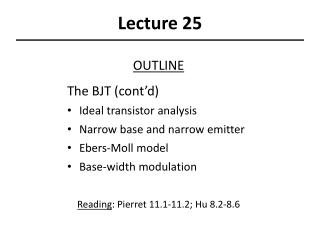DownloadDownload PresentationLecture 25

# Lecture 25

Download Presentation## Lecture 25

- - - - - - - - - - - - - - - - - - - - - - - - - - - E N D - - - - - - - - - - - - - - - - - - - - - - - - - - -
##### Presentation Transcript

1. Lecture 25 OUTLINE The BJT (cont’d) • Ideal transistor analysis • Narrow base and narrow emitter • Ebers-Moll model • Base-width modulation Reading: Pierret 11.1-11.2; Hu 8.2-8.6

2. Notation (PNP BJT) NENAE DEDN tE tn LELN nE0 np0 = ni2/NE NBNDB DBDP tB tp LBLP pB0 pn0ni2/NB NCNAC DCDN tC tn LCLN nC0 np0ni2/NC EE130/230M Spring 2013 Lecture 25, Slide 2

3. “Game Plan” for I-V Derivation • Solve the minority-carrier diffusion equation in each quasi-neutral region to obtain excess minority-carrier profiles • different set of boundary conditions for each region • Find minority-carrier diffusion currents at depletion region edges • Add hole & electron components together  terminal currents EE130/230M Spring 2013 Lecture 25, Slide 3

4. Emitter Region Analysis • Diffusion equation: • General solution: • Boundary conditions: • Solution: EE130/230M Spring 2013 Lecture 25, Slide 4

5. Collector Region Analysis • Diffusion equation: • General solution: • Boundary conditions: • Solution: EE130/230M Spring 2013 Lecture 25, Slide 5

6. Base Region Analysis • Diffusion equation: • General solution: • Boundary conditions: • Solution: EE130/230M Spring 2013 Lecture 25, Slide 6

7. Since we can write as EE130/230M Spring 2013 Lecture 25, Slide 7

8. EE130/230M Spring 2013 Lecture 25, Slide 8

9. BJT Terminal Currents • We know: • Therefore: EE130/230M Spring 2013 Lecture 25, Slide 9

10. BJT with Narrow Base • In practice, we make W << LB to achieve high current gain. Then, since we have: EE130/230M Spring 2013 Lecture 25, Slide 10

11. BJT Performance Parameters • Assumptions: • emitter junction forward biased, collector junction reverse biased • W << LB EE130/230M Spring 2013 Lecture 25, Slide 11

12. BJT with Narrow Emitter Replace with WE’ if emitter is narrow EE130/230M Spring 2013 Lecture 25, Slide 12

13. Ebers-Moll Model increasing (npn) or VEC (pnp) • The Ebers-Moll model is a large-signal equivalent circuit which describes both the active and saturation regions of BJT operation. • Use this model to calculate IB and IC given VBE and VBC EE130/230M Spring 2013 Lecture 25, Slide 13

14. V V EB CB I B E B C I C If only VEB is applied (VCB = 0): aR : reverse common base gain aF : forward common base gain If only VCBis applied (VEB = 0): : Reciprocity relationship: EE130/230M Spring 2013 Lecture 25, Slide 14

15. In the general case, both VEB and VCB are non-zero: IC: C-B diode current + fraction of E-B diode current that makes it to the C-B junction IE: E-B diode current + fraction of C-B diode current that makes it to the E-B junction Large-signal equivalent circuit for a pnp BJT EE130/230M Spring 2013 Lecture 25, Slide 15

16. Base-Width Modulation Common Emitter Configuration, Active Mode Operation W IE IC P+ N P  + VEB DpB(x) IC (VCB=0) x VEC W(VBC) 0 EE130/230M Spring 2013 Lecture 25, Slide 16

17. Ways to Reduce Base-Width Modulation Increase the base width, W Increase the base dopant concentration NB Decrease the collector dopant concentration NC Which of the above is the most acceptable action? EE130/230M Spring 2013 Lecture 25, Slide 17

18. Early Voltage, VA Output resistance: A large VA (i.e. a large ro ) is desirable IC IB3 IB2 IB1 VEC 0 VA EE130/230M Spring 2013 Lecture 25, Slide 18

19. Derivation of Formula for VA Output conductance: for fixed VEB where xnC is the width of the collector-junction depletion region on the base side xnC P+ N P EE130/230M Spring 2013 Lecture 25, Slide 19

20. EE130/230M Spring 2013 Lecture 25, Slide 20

21. Summary: BJT Performance Requirements • High gain (bdc >> 1) • One-sided emitter junction, so emitter efficiency g  1 • Emitter doped much more heavily than base (NE >> NB) • Narrow base, so base transport factor aT 1 • Quasi-neutral base width << minority-carrier diffusion length (W << LB) • IC determined only by IB (IC function of VCE,VCB) • One-sided collector junction, so quasi-neutral base width W does not change drastically with changes in VCE (VCB) • Based doped more heavily than collector (NB > NC) (W = WB – xnEB – xnCB for PNP BJT) EE130/230M Spring 2013 Lecture 25, Slide 21